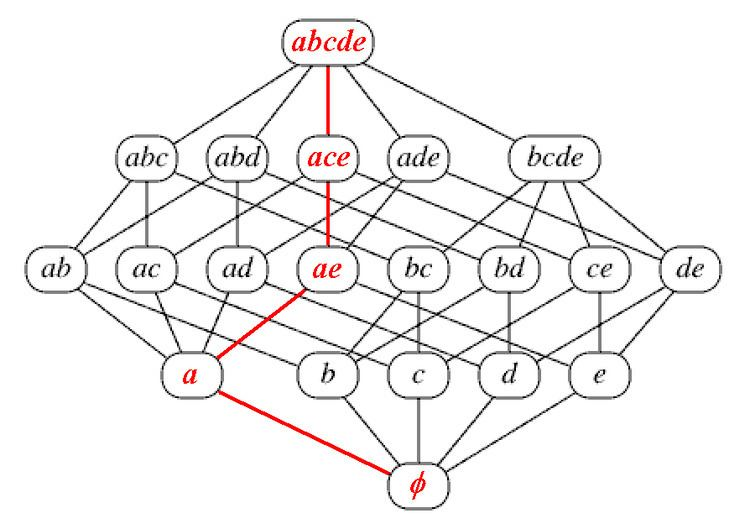# Flag (geometry)

Updated on
Covid-19In (polyhedral) geometry, a flag is a sequence of faces of a polytope, each contained in the next, with just one face from each dimension.

More formally, a flag ψ of an n-polytope is a set {F−1, F0, ..., Fn} such that FiFi+1 (−1 ≤ in − 1) and there is precisely one Fi in ψ for each i, (−1 ≤ in). Since, however, the minimal face F−1 and the maximal face Fn must be in every flag, they are often omitted from the list of faces, as a shorthand. These latter two are called improper faces.

For example, a flag of a polyhedron comprises one vertex, one edge incident to that vertex, and one polygonal face incident to both, plus the two improper faces. A flag of a polyhedron is sometimes called a "dart".

A polytope may be regarded as regular if, and only if, its symmetry group is transitive on its flags. This definition excludes chiral polytopes.

## Incidence geometry

In the more abstract setting of incidence geometry, which is a set having a symmetric and reflexive relation called incidence defined on its elements, a flag is a set of elements that are mutually incident. This level of abstraction generalizes both the polyhedral concept given above as well as the related flag concept from linear algebra.

A flag is maximal if it is not contained in a larger flag. When all maximal flags of an incidence geometry have the same size, this common value is the rank of the geometry.

## References

Similar Topics
My Giant
Pierre Strauch
Alfonso I of Asturias
Topics